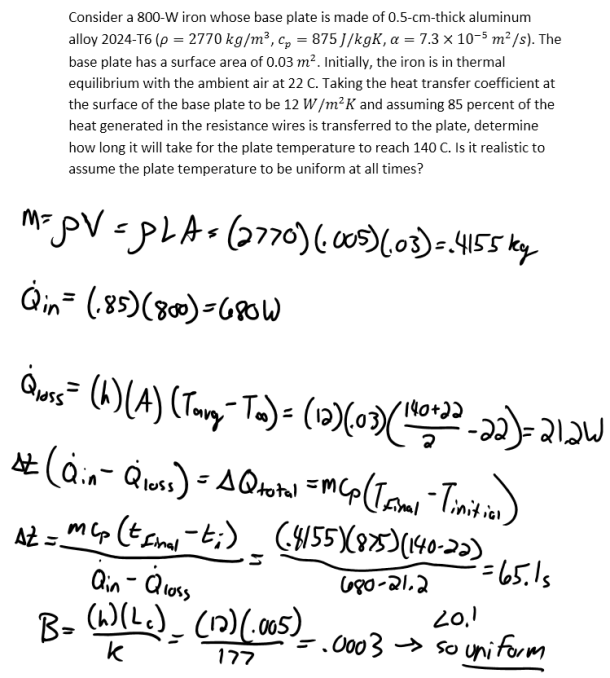heat and mass transfer problems engineering equations heat and mass transfer school homework engineering heat and mass transfer formulas heat and mass transfer solutions to heat and mass transfer problems full solution engineering problem solution heat and mass transfer math problems engineering equations heat and mass transfer school homework engineering solutions to heat and mass transfer formulas heat problems mass problem solutions to transfer problems full solution heat and mass transfer
heat and mass transfer problems engineering equations heat and mass transfer school homework engineering heat and mass transfer formulas heat and mass transfer solutions to heat and mass transfer problems full solution engineering problem solution heat and mass transfer math problems engineering equations heat and mass transfer school homework engineering solutions to heat and mass transfer formulas heat problems mass problem solutions to transfer problems full solution heat and mass transfer
Highalphabet Home Page heat and mass transfer problem solutions Heat and Mass Transfer Page
Consider a 800-W iron whose base plate is made of 0.5-cm-thick aluminum alloy 2024-T6 (rho=2770 kg/m^3, c_p=875 J/kgK, alpha=7.3×10^(-5) m^2/s). The base plate has a surface area of 0.03 m^2. Initially, the iron is in thermal equilibrium with the ambient air at 22 C. Taking the heat transfer coefficient at the surface of the base plate to be 12 W/m^2 K and assuming 85 percent of the heat generated in the resistance wires is transferred to the plate, determine how long it will take for the plate temperature to reach 140 C. Is it realistic to assume the plate temperature to be uniform at all times?Consider a 800-W iron whose base plate is made of 0.5-cm-thick aluminum alloy 2024-T6 (rho=2770 kg/m^3, c_p=875 J/kgK, alpha=7.3×10^(-5) m^2/s). The base plate has a surface area of 0.03 m^2. Initially, the iron is in thermal equilibrium with the ambient air at 22 C. Taking the heat transfer coefficient at the surface of the base plate to be 12 W/m^2 K and assuming 85 percent of the heat generated in the resistance wires is transferred to the plate, determine how long it will take for the plate temperature to reach 140 C. Is it realistic to assume the plate temperature to be uniform at all times?